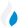•Trade Pi
•Trade
•Exchange
•Trade Pi
•Trade
•Exchange
\$1.13T
Total marketcap
\$91.32B
Total volume
40.62%
BTC dominance
•Trade Pi
•Trade
•Exchange

# TRX to BTR Exchange Rate - 1 TRON in Bitrue Coin

1.793447
• BTC 0.0000027
• ETH 0.000038
Vol [24h]
\$150,238.92

## trx to btr converter

Exchange Pair Price 24h volume
Bitrue TRX/BTR \$0.0646 \$152.02K

## TRX/BTR Exchange Rate Overview

Name Ticker Price % 24h 24h high 24h low 24h volume
TRON trx \$0.06418 2.801% \$0.06419 \$0.06144 \$387.82M
Bitrue Coin btr \$0.03566 7.8758% \$0.03635 \$0.03244 \$5.42M

Selling 1 TRX you get 1.793447 Bitrue Coin btr.

TRON Jan 5, 2018 had the highest price, at that time trading at its all-time high of \$0.2316.

1854 days have passed since then, and now the price is 27.68% of the maximum.

Based on the table data, the TRX vs BTR exchange volume is \$150,238.92.

Using the calculator/converter on this page, you can make the necessary calculations with a pair of TRON/Bitrue Coin.

## Q&A

### What is the current TRX to BTR exchange rate?

Right now, the TRX/BTR exchange rate is 1.793447.

### What has been the TRON to Bitrue Coin trading volume in the last 24 hours?

Relying on the table data, the TRON to Bitrue Coin exchange volume is \$150,238.92.

### How can I calculate the amount of BTR? / How do I convert my TRON to Bitrue Coin?

You can calculate/convert BTR from TRON to Bitrue Coin converter. Also, you can select other currencies from the drop-down list.

## TRX to BTR Сonversion Table

TRX BTR
8 TRX = 14.3 BTR
20 TRX = 35.8 BTR
40 TRX = 71.7 BTR
50 TRX = 89.6 BTR
80 TRX = 143.4 BTR
200 TRX = 358.6 BTR
300 TRX = 538.03 BTR
800 TRX = 1,434.7 BTR
2000 TRX = 3,586.8 BTR
20000 TRX = 35,868.9 BTR
200000 TRX = 358,689.4 BTR
2000000 TRX = 3,586,894 BTR
20000000 TRX = 35,868,940 BTR